# Retrieving the Nth Value in a Row, Column and Range in Microsoft Excel 2010

In this article, we will learn how to retrieve the Nth value in a Row, Column, and range. We use a combination of “OFFSET”, “Index”, and “ROW” functions in Microsoft Excel 2010.

OFFSET: -One of the rows and columns in a range that is a specified number of the cells or range of cells has a reference returned references. A single cell or range of cells, you may have to be returned to the number of rows and number of columns, can be specified.

The syntax of OFFSET function:    =OFFSET (reference, rows, cols, height, width)

Reference:- This is a cell or range from which you want to offset.

Rows and Columns to move: - How many rows you want to move from the starting point, and both of these can be positive, negative or zero.

Height and Width: - This is the size of the range you want to return.

Let’s take an example to understand how the Offset function works in excel.

We have data in range A1:D10 in which column A contains Product Code, Column B contains Quantity, column C contains per product cost and column D contains Total cost. We want to return the value of cell C5 in cell E2.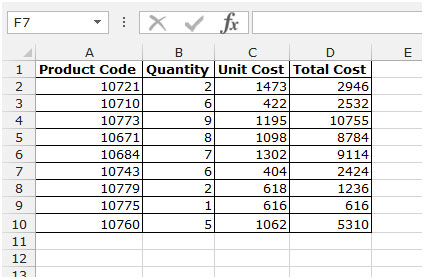• Select the cell E2 and write the formula.
• =OFFSET(A1,4,2,1,1)
• Press Enter on your keyboard.
• The function will return the value of cell C5.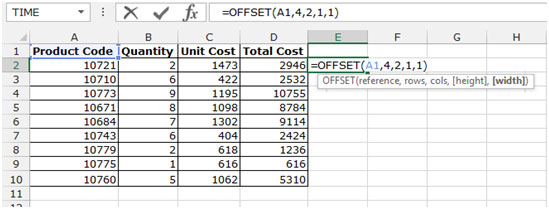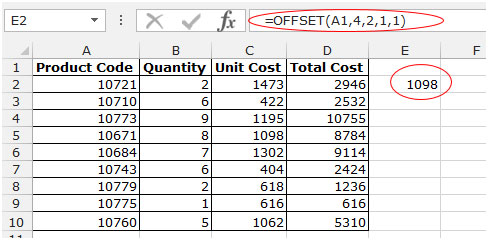ROW: - This function is used to return the row number of a cell reference.

Syntax of “ROW” function:           =ROW (reference)

Example: =ROW (A1) would return 1

=ROW (A1:A10) would return 1

=ROW (A1:C10) would return 1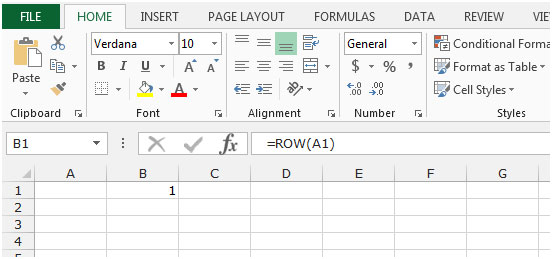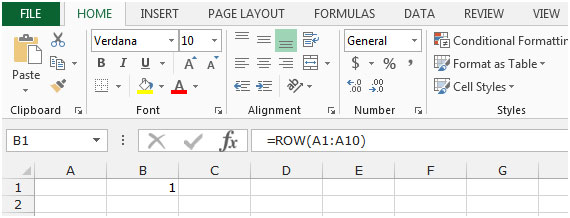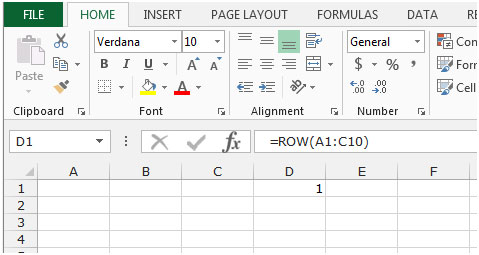INDEX: The Index formula returns a value from the intersection between the row number and the column number in an Array. There are 2 syntaxes for the “INDEX” function.

1st Syntax of “INDEX” function:=INDEX (array, row_num, [column_num])

2nd Syntax of “INDEX” function:=INDEX (reference, row_num, [column_num], [area_num])

For example, we have data in range A1:C5 in which column A contains Order Id, column B contains Unit Price, and column C contains Quantity.

• Write the formula in cell D2.
• =INDEX(A1:C5,2,3)
• Press Enter on your keyboard.
• The function will return 10, which means 10 is available in 2nd row and 3rd column.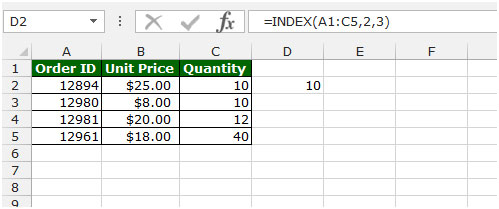Let’s take an example to understand how we can retrieve the Nth value in a row, column and range.

We have data in range A1:B12.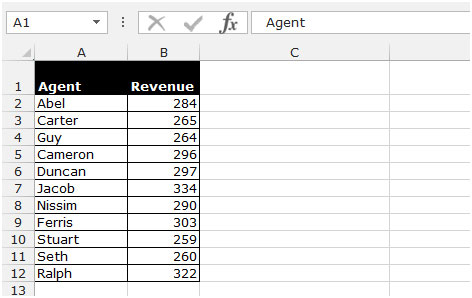To retrieve the Nth value in the column, follow below given steps:-

• Write the formula in cell C2.
• =OFFSET(\$B\$1,0,C4-1)
• Press Enter on your keyboard.
• The function will return the Nth value in the column.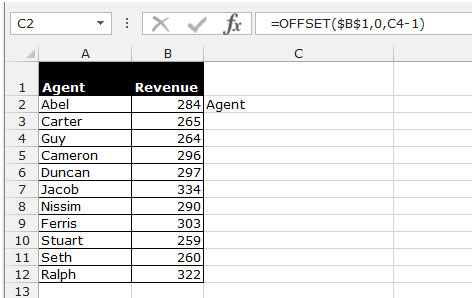To retrieve the Nth value in the Row, follow below given steps:-

• Write the formula in cell C2.
• =OFFSET(\$A\$4,C4-1,0)
• Press Enter on your keyboard.
• The function will return the Nth value in the row.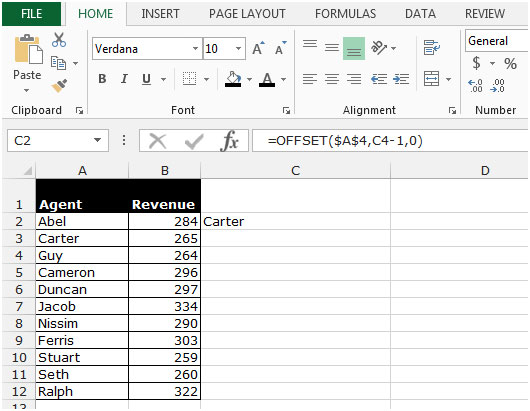To retrieve the Nth value in the Range, follow below given steps:-

• Write the formula in cell C2.
• =INDEX(\$A\$2:\$A\$12,(ROW()-2)*3+3)
• Press Enter on your keyboard.
• The function will return the Nth value in the range.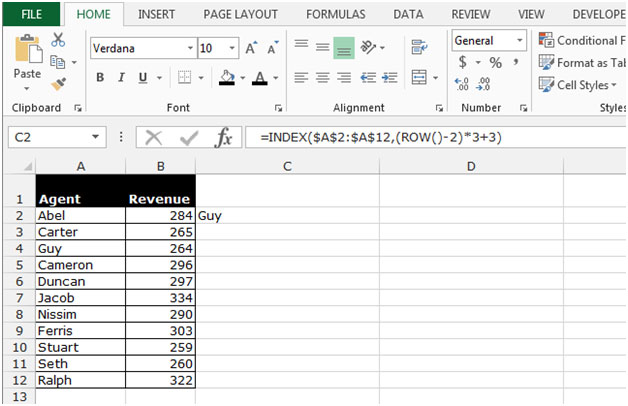This is the way we can retrieve the Nth value in a row, column, and range in Microsoft Excel 2010.

1.Column 1& 2 Unique value can be manually entered. I want the numbers laid by the excel function from the list wrt each row and column as display

Column 1 Column 2 Column 3
A W 1
A X 2
A Y 3
A Z 4
B W 5
B X 6
B Y 7
B Z 8
C W 9
C X 10
C Y 11
C Z 12
D W 13
D X 14
D Y 15
D Z 16

W X Y Z
A 1 2 3 4
B 5 6 7 8
C 9 10 11 12
D 13 14 15 16

Terms and Conditions of use

The applications/code on this site are distributed as is and without warranties or liability. In no event shall the owner of the copyrights, or the authors of the applications/code be liable for any loss of profit, any problems or any damage resulting from the use or evaluation of the applications/code.